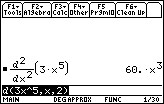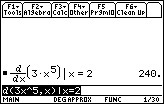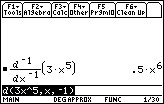# Knowledge Base

## Solution 17169: Calculating the Second Derivative and Antiderivative Using a TI-89 Family, TI-92 Family and Voyage™ 200 Graphing Calculator.

### How do I calculate the second derivative and antiderivative using a TI-89 family, TI-92 family or Voyage 200 calculator?

The differentiate command, d (differentiate), computes derivatives.

Please see the below example for computing the second derivative:

Example: What is the second derivative of 3x5?

1) Press [F3] to select 1:d( differentiate.
2) Enter 3x^5) and press [ENTER].Please Note: It is also possible to take the derivative of a previous answer with d(ans(1),x) when the answer is recalled.

To evaluate a derivative at a specific value, use the with bar ( | ). On the Voyage 200, press [2nd][K]. Evaluate 3x when x=2.

1) Enter 3x^5,x)|x=2 and press [ENTER].To find the antiderivative, enter d (3x^5,x,-1).Please see the TI-89 family, TI-92 family and Voyage 200 guidebooks for additional information.